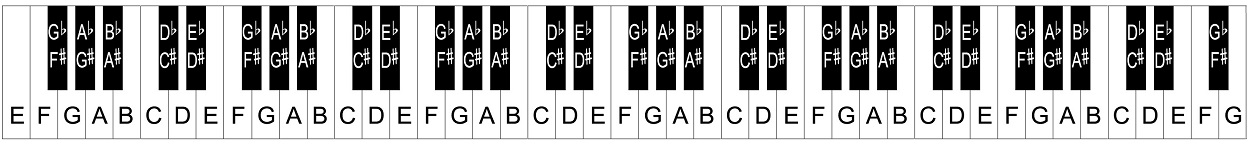# How can I calculate the Number of bins per octave?

I am trying to calculate the Constant Q Transform for Monophonic audio using Python. The Python has the library to calculate the CQT , the default value of num_bins_per_octave is 12.

How would one decide the number bins? Newbie to the Topic. Please enlighten me.

• I don't know how to choose the optimum number of bins for all applications, but I can tell that the default value 12 is most probably due to the fact that the most-widely used tuning system for western music uses twelve tones per octave. Jan 17 '19 at 10:02
• Thanks alot! But how about number of bins? Is it 84 always , because the default values says so Jan 17 '19 at 11:15
• Please let me know if i have to ask new question for this! As this is new query again Jan 17 '19 at 11:24
• 84 bins correspond to 7 octaves as $\tfrac{84}{12}=7$. This makes sense as a default value because the note range of most of the typical music instruments is captured then. To give more hints, could you tell me which Python library are you using? Jan 17 '19 at 12:06
• I'm using librosa librosa.github.io/librosa/generated/librosa.core.cqt.html Jan 17 '19 at 12:27

I do not know how to determine the ideal number of bins. However, I can shed some light on why in Librosa's CQT function, the default values are num_bins_per_octave=12 and n_bins=84. (This is my first answer so any feedback would be helpful. And because this does not directly answer the question, should I perhaps post this as a comment?)

The Librosa CQT function you mention can be found in the Librosa documentation here.

## First some music theory

Western music consists of 12 identifiable types of pitches. Each of these 12 pitches is considered a new note. The 12 pitches are as follows:

C
C# / Db
D
D# / Eb
E
F
F# / Gb
G
G# / Ab
A
A# / Bb
B

The notes with slashes can be called by either name, depending upon which key we’re in. (It doesn't matter what a key is right now.)

These 12 pitches repeat in the same order throughout the complete span of human hearing. So after B, there would be another C, then another C#, another D, and so on and so forth, giving us multiple C pitches, C# pitches, D pitches, etc.

A specific group of these 12 pitches is defined by something called an octave. An octave is the distance between one note (like C#) and the next note bearing its same name (the next C# that’s either higher or lower).

The reason why an octave is divided into 12 pitches rather than, let's say 11 or 13 pitches, can be found here.

Librosa's default num_bins_per_octave is 12 specifically because there are 12 pitches in an octave.

## The piano

A great visual of multiple consecutive octaves can be seen in this 76-key piano here:Notice that the set of 12 unique pitches repeats over and over again on this keyboard as you move left to right (or vice versa), counting both the black and white keys.

A normal piano has 88-keys, 7 octaves plus four extra notes. Looking at the 7 octaves, each octave has 12 pitches giving us a total of 7*12=84 pitches on this part of the piano. This is where the 84 from in the n_bins=84 parameter of the Librosa CQT method comes from.

There are multiple reasons why the standard piano has 88 keys instead of 84 that you can read about here.

Hope this helps.